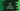# Java program to sort array elements by frequency## Java program to sort elements by frequency:

In this post, we will learn how to sort an array of numbers by frequency. We will sort the numbers in descending order. For example, we have the array [1, 2, 3, 4, 3, 3, 2], then the program will return array [3, 3, 3, 2, 2, 1, 4], which holds all numbers sorted based on their frequencies.

Also, note that if more than one numbers are appearing for equal number of times, then it will print the number which comes first.

### Algorithm:

We need to maintain the number of occurrences for each element in the array. We can do that by using a LinkedHashMap. The advantage of LinkedHashMap is that it maintains the insertion order.

So, the program will iterate over the numbers in the array one by one, and it will keep the occurrence count of each number in the LinkedHashMap. If two numbers have the same frequency, LinkedHashMap will put them keeping their order of occurrence in the array.

Once all items are iterated, the LinkedHashMap will hold the occurrence count for each number. At this point, we can sort it by considering the values.

For sorting, we can use Collections.sort() method and pass our own comparator method.

## Java program:

Let’s take a look at the program:

``````package com.company;

import java.util.*;
import java.util.stream.Collectors;

public class Main {

return givenHashMap.entrySet()
.stream()
.sorted(Collections.reverseOrder(Map.Entry.comparingByValue()))
.collect(Collectors.toMap(
Map.Entry::getKey,
Map.Entry::getValue,
}

private static Integer[] getSortedArray(Integer[] arr) {

for (Integer value : arr) {
if (map.containsKey(value)) {
map.put(value, map.get(value) + 1);
} else {
map.put(value, 1);
}
}

Map<Integer, Integer> sortedMap = sortByValue(map);

ArrayList<Integer> sortedList = new ArrayList<>();

sortedMap.forEach((key, value) -> {
for (int i = 1; i <= value; i++) {
}
});

return sortedList.toArray(new Integer);
}

public static void main(String[] args) {
System.out.println(Arrays.toString(getSortedArray(new Integer[]{5, 4, 3, 2, 1, 1, 1, 2, 3, 3, 3, 4, 9})));
}
}``````

Here,

• getSortedArray method is used to sort an array based on the frequency of the elements. This program is using an integer array, but you can also modify it to any other types.
• In getSortedArray,
• First of all, it created one LinkedHashMap called map.
• It is then iterating through the items of the array arr one by one and updating the frequencies in the LinkedHashMap. If we have any value, it is updating the value, i.e. adding 1 to it. Else, it is setting 1 to the value.
• sortedMap is the sorted map, i.e. the LinkedHashMap we created above sorted by the frequencies.
• It calls sortByValue method that sorts the LinkedHashMap based on the frequencies.
• sortedList is an empty arraylist, where we are adding all final data.
• We are looping through the sortedMap and adding the values from the map to the array list.
• It converts the list to array and returns that value.

## Output:

If you run this program, it will print the below output:

``[3, 3, 3, 3, 1, 1, 1, 4, 4, 2, 2, 5, 9]``

As you can see here, it sorts the array based on the frequency of each item. 4 and 2 occurred two times each. But, since 4 is before 2 in the array, it puts 4, 4 before 2, 2.